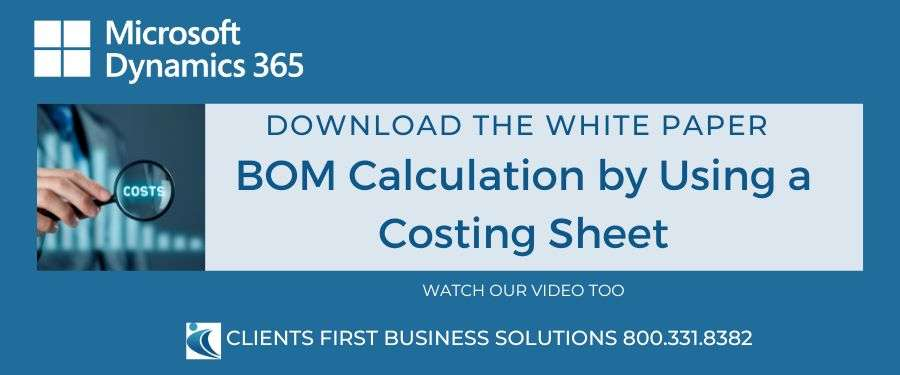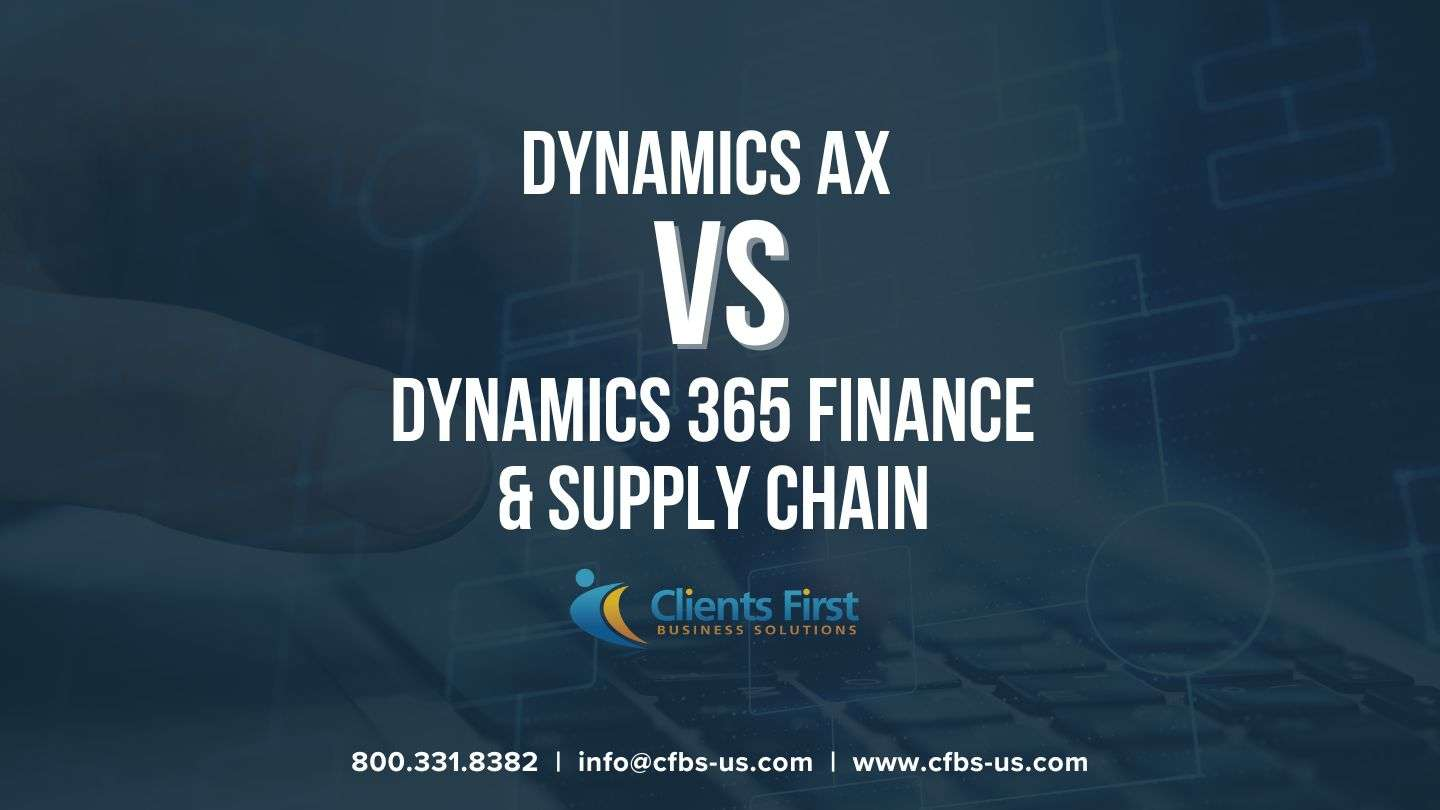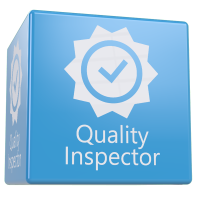Microsoft Dynamics 365 Supply Chain simplifies absorption costing and Bill Of Material (BOM) calculations, ensuring precise product costs and inventory valuation. With features like cost groups, costing versions, and integration with production orders, businesses can efficiently track and manage costs throughout manufacturing.

# Understanding BOM Calculations and Absorption Costing with Microsoft Dynamics 365 Supply Chain

BOM calculations and absorption costing are vital components for accurately determining product costs and valuing inventory in the manufacturing industry. Microsoft Dynamics 365 Supply Chain provides robust support for these processes, enabling businesses to effectively absorb indirect costs into product costs. This article offers a concise overview of how Dynamics 365 Supply Chain (formerly Dynamics 365 for Operations) facilitates absorption costing and BOM calculations.

## Absorption Costing and Its Importance

Absorption costing ensures that indirect costs arising from the production of finished goods are recognized and incorporated into product costs. In industries where indirect costs constitute a significant portion of overall expenses, accurate absorption is crucial for precise product costing and inventory valuation.

## Cost Groups and Costing Versions

Dynamics 365 Supply Chain utilizes cost groups and costing versions to streamline absorption costing. Cost groups serve as the foundation of cost composition, categorizing resources into different types such as direct material, direct manufacturing, indirect cost, and direct outsourcing. On the other hand, costing versions serve as repositories for cost records for items and cost categories, determining current active costs and facilitating BOM calculations.

## The Costing Sheet

The costing sheet in Dynamics 365 Supply Chain plays a vital role in displaying cost information and incorporating overhead costs. It consists of nodes representing totals and subtotals, structured based on the cost group list. Various types of nodes, including price nodes, total nodes, and cost group nodes, enable effective grouping and calculation of costs. Indirect cost sub-nodes, such as surcharges, rates, output unit-based, and input unit-based nodes, allow for precise calculation of indirect costs.

## Key Steps in Implementing Absorption Costing and BOM Calculations

The key steps involved in implementing absorption costing and BOM calculations using Microsoft Dynamics 365 Supply Chain include:

• Setting up cost groups - Create and configure groups that categorize items or resources based on similar cost structures for better cost tracking and analysis.
• Assigning cost groups to items and cost categories - Associate the defined cost groups with specific items and cost categories to establish the relationship between cost structures and elements within your organization.
• Configuring costing versions - Define different sets of cost standards or rules, such as standard or actual costing, by configuring costing versions to determine how costs are calculated and assigned to items or resources.
• Defining the costing sheet format  - Specify the sequence and structure of cost calculations by setting up the costing sheet format and determining how costs are accumulated, rolled up, and assigned based on defined cost groups, cost categories, and costing versions.

## Calculating a BOM by Using Single-level and Multi-level Explosions

Single-level explosion focuses on immediate subcomponents, disregarding lower-level components or subassemblies. It begins with the top-level product, identifies its direct components, and includes quantities. If subcomponents have their own BOMs, the process repeats.

Multi-level explosion expands the BOM to include all levels of subcomponents and subassemblies. It recursively applies the same steps, ensuring a comprehensive breakdown of components. Quantities are aggregated to determine the total required. The choice depends on the desired level of detail and complexity needed for the BOM.

## Indirect Costs and Costing Sheet Setup

In addition to single-level and multilevel BOM calculations, various types of indirect costs need to be considered in the costing process. These indirect costs are categorized into four types: surcharge, rate, output unit-based, and input unit-based.

To calculate indirect costs, you need to set up a costing sheet that defines the cost structure. The costing sheet includes nodes and subnodes representing different cost groups and their calculations. Let's explore the setup process for some of the indirect cost nodes:

### Surcharge Node

The surcharge is an indirect cost calculated as a percentage applied to raw materials or operational processes. Follow these steps to set up a surcharge node:

1. Open the Costing Sheet Setup page.
2. Select the sub-node under the Material Overhead node, such as "Internal logistics - % of cost."
3. Set the subtype (Level, Sublevel, or Total) based on your calculation requirements.
4. Configure the absorption basis, such as selecting the material nodes for applying the surcharge.
5. Add formulas to calculate the surcharge, specifying the costing version, site, percentage, and effective date.
6. Define ledger postings and financial dimensions for the indirect cost.

### Rate Node

The rate is an indirect cost calculated as a constant amount added to the operational process. Follow these steps to set up a rate node:

1. Select the sub-node under the Labor Overhead node, such as "Indirect labor cost - Rate per process time."
2. Set the subtype (Process, Setup, or Quantity) based on the cost allocation method.
3. Configure the absorption basis, such as selecting the manufacturing nodes to apply the rate.
4. Add formulas to calculate the rate, specifying the costing version, site, amount, and effective date.
5. Define ledger postings and financial dimensions for the indirect cost.

### Output Unit-Based Node

The output unit-based indirect cost is applied for each unit produced and based on quantity, weight, or volume. Follow these steps to set up an output unit-based node:

1. Select the sub-node under the Plant Overhead node, such as "Machine depreciation - Output unit based."
2. Set the subtype (Quantity, Weight, or Volume) depending on the measurement basis.
3. Configure the absorption basis, if applicable.
4. Add formulas to calculate the rate, specifying the costing version, site, amount, and effective date.
5. Define ledger postings and financial dimensions for the indirect cost.

### Input Unit-Based Node

The input unit-based indirect cost is applied for each unit of income material (raw material) and is based on weight or volume. Follow these steps to set up an input unit-based node:

1. Create a new node under the Plant Overhead node with the Input unit-based type.
2. Define the subtype (Weight or Volume) based on the measurement basis.
3. Configure the absorption basis, such as selecting the manufacturing nodes to which the cost applies.
4. Add formulas to calculate the rate, specifying the costing version, site, amount, and effective date.
5. Define ledger postings and financial dimensions for the indirect cost.

The inclusion of these indirect cost nodes in the costing sheet unveils an efficient mechanism to calculate and assign costs across a multitude of cost groups and subgroups, delivering a comprehensive overview of the cost structure.

## The Impact of Subtype Field Value on Surcharge Calculation Results

Different subtypes in surcharge, rate, output unit-based, and input unit-based calculations behave differently on the Costing Sheet Setup page. Here's a summary of their behaviors:

### Surcharge Subtype Behavior

• Total Subtype: The surcharge is cumulated per level and applied to the total cost of the level.
• Level Subtype: The surcharge is applied individually for each level, applying to the corresponding amount at each level.
• Sublevel Subtype: The surcharge is added only at the first level and applied to the value of that level, including any levels below it.

### Rate Subtype Behavior

• Process Subtype: The rate is added per unit of process time (run time) for each level.
• Setup Subtype: The rate is added per unit of setup time for each level.
• Quantity Subtype: The rate is added per unit of processed quantity for each level.

### Output Unit-Based Subtype Behavior

• Quantity Subtype: An amount is added per unit of produced quantity for each level.
• Weight Subtype: An amount is added per unit of produced weight for each level.
• Volume Subtype: An amount is added per unit of produced volume for each level.

### Input Unit-Based Subtype Behavior

• Volume Subtype: An amount is added per unit of income volume for each level.
• Weight Subtype: An amount is added per unit of income weight for each level.

Unlocking the secrets behind these subtypes' behavior empowers businesses to accurately compute costs and allocate them based on factors like total cost, level-wise cost, process time, setup time, quantity, weight, or volume. This information plays a crucial role in analyzing cost structures and making informed decisions regarding pricing and profitability.

## Indirect Cost Calculation from a Production Order

To calculate indirect costs from a production order, follow these steps:

### 1. Activate a price for the main BOM:

1. Open the Released products page and select BOM_1.
2. On the Manage Costs tab of the Action Pane, click Item Price.
3. Delete any pending prices.
4. Run item calculation using a single explosion.
5. Click Activate pending price(s).

### 2. Add stock for the raw material:

1. Open the Inventory adjustment journal page.
2. Create a journal header for site 1 and warehouse 11.
3. Add three journal lines with the following items and quantities:
• ITEM_A: 10
• ITEM_B: 10
• BOM_2: 10
1. Click Post when finished.

### 3. Create and process a production order:

1. Open the All production orders page.
2. Click New production order.
3. Enter the item to produce (BOM_1), quantity (1), site (1), and warehouse (11).
4. When asked to insert the active BOM and route, click Yes.
5. Click Create when finished.
6. On the Production order tab of the Action Pane, click Estimate, then click OK.

### 4. View the results of a BOM calculation from a production order:

1. Select the estimated production order.
2. On the Manage Costs tab of the Action Pane, click View calculation details.
3. The Price calculation page displays the structure based on the costing sheet but at the production order level.
4. The Overview estimation tab shows details of estimated costs based on the costing sheet structure and active costs, calculated at a single level.
5. Switch to the Overview costing tab to view actual and estimated costs as the production order progresses.
6. Use the Costing sheet tab to examine the data within the costing sheet structure.
7. Apply filters in the Level, Per, and Mode fields to customize the view of costs.

### 5. View indirect cost transactions of a production order:

1. Go back to the production order.
2. On the Manage Costs tab of the Action Pane, click Production Posting.
3. On the Production posting page, click Indirect cost transactions.
4. The Indirect cost transactions page displays details of the indirect costs posted for the production order.
5. At this stage, only work-in-progress (WIP) postings have been performed.
6. Financial values and vouchers will appear once the production order is completed.

## Conclusion

Microsoft Dynamics 365 Supply Chain provides robust support for BOM calculation and absorption costing, crucial processes for accurate product cost determination, and inventory valuation in manufacturing. The software enables effortless allocation of indirect costs into product costs through cost groups, costing versions, and the costing sheet.

Various indirect cost nodes, including surcharge, rate, output unit-based, and input unit-based, ensure precise cost calculation and allocation across cost groups. Integration with production orders allows for tracking and analysis of costs at each manufacturing stage, facilitating pricing and profitability decisions. Microsoft Dynamics 365 Supply Chain streamlines BOM calculations and absorption costing, resulting in improved cost management and operational efficiency.

If you're interested in learning more about the Dynamics 365 Finance and Supply Chain be sure to contact Clients First today!#### Dynamics AX vs Dynamics 365 Finance and Supply Chain

When it comes to Dynamics AX 2009, AX 2012, and AX 2012 R3, customers are faced with a crucial decision: stick with their legacy platform or make the...#### Exploring the Business Central Modules: A Comprehensive Overview

Explore the extensive range of modules offered by Business Central and discover how they can contribute to the success of your business. Microsoft...#### Quality Control Software for Dynamics 365 Business Central

Integrated Quality Control Software to Simplify and Automate Quality Control Processes Insight Works Quality Inspector app simplifies and automates...